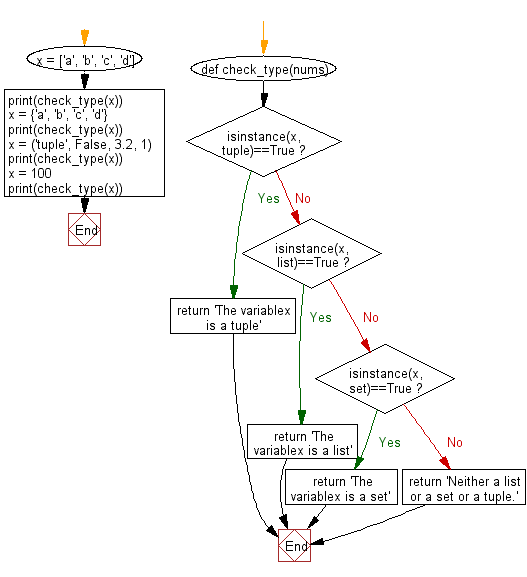﻿ Python: Test if a variable is a list or tuple or a set - w3resource# Python: Test if a variable is a list or tuple or a set

## Python Basic: Exercise-145 with Solution

Write a Python program to test if a variable is a list or tuple or a set.

Sample Solution-1:

Python Code:

``````#x = ['a', 'b', 'c', 'd']
#x = {'a', 'b', 'c', 'd'}
x = ('tuple', False, 3.2, 1)
if type(x) is list:
print('x is a list')
elif type(x) is set:
print('x is a set')
elif type(x) is tuple:
print('x is a tuple')
else:
print('Neither a list or a set or a tuple.')
``````

Sample Output:

```x is a tuple
```

## Visualize Python code execution:

The following tool visualize what the computer is doing step-by-step as it executes the said program:

Sample Solution-2:

Python Code:

``````def check_type(nums):
if isinstance(x, tuple)==True:
return 'The variablex is a tuple'
elif isinstance(x, list)==True:
return 'The variablex is a list'
elif isinstance(x, set)==True:
return 'The variablex is a set'
else:
return 'Neither a list or a set or a tuple.'
x = ['a', 'b', 'c', 'd']
print(check_type(x))
x = {'a', 'b', 'c', 'd'}
print(check_type(x))
x = ('tuple', False, 3.2, 1)
print(check_type(x))
x = 100
print(check_type(x))
``````

Sample Output:

```The variablex is a list
The variablex is a set
The variablex is a tuple
Neither a list or a set or a tuple.
```

Flowchart:## Visualize Python code execution:

The following tool visualize what the computer is doing step-by-step as it executes the said program:

Python Code Editor :

Have another way to solve this solution? Contribute your code (and comments) through Disqus.

What is the difficulty level of this exercise?

Test your Python skills with w3resource's quiz

﻿

## Python: Tips of the Day

Unpack variables from iterable:

```# One can unpack all iterables (tuples, list etc)
>>> a, b, c = 1, 2, 3
>>> a, b, c
(1, 2, 3)

>>> a, b, c = [1, 2, 3]
>>> a, b, c
(1, 2, 3)
```